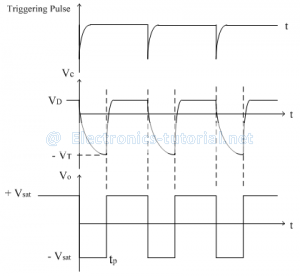# Monostable multivibrator

In this multivibrator one state is permanent stable state while the other state is temporary state. The permanent stable state is either HIGH or LOW. Assume the permanent state is HIGH. Apply a triggering pulse to change output state to LOW. But this state is a temporary state. So it will remain in LOW state for some time and after some time without applying any triggering pulse output goes back to permanent stable state i.e. HIGH.
Thus only one triggering pulse is required to come back to the permanent stable state so frequency of output is equal to frequency of input triggering pulse. Thus called as single shot or mono-shot multivibrator. The following circuit diagram shows monostable multivibrator using op-amp.The triggering pulses are obtained by differentiating a square wave. The pulse has large amplitude and small width. In most of the circuits we require only one type of pulses either positive or negative. So one type of pulses should be eliminated. This is done with the help of diode when it is forward biased.
Let us assume, for above circuit the stable state is +Vsat. so diode D is forward biased and voltage across is 0.7V. Thus voltage across capacitor (i.e. voltage at inverting terminal) is 0.7V.
Select values of R1 and R2 such that voltage an non-inverting terminal is greater than 0.7V so output is +Vsat. Voltage across capacitor is always 0.7V because of diode. In forward biased condition resistance of diode is very small so capacitor is almost short so no charging takes place.
If output want to be –Vsat, then voltage at inverting terminal should be greater than non-inverting terminal. So apply a negative triggering pulse at non-inverting terminal to change the output state.
Working:
A negative triggering pulse is applied at t = 0. Initially drop across capacitor C is 0.7V and output is +Vsat. When pulse is applied at t = 0, output state changes to -Vsat. The diode D is reversed biased so capacitor starts charging through resistance R towards-Vsat. When output is –Vsat, triggering point VT is also negative, so when capacitor charges upto –VT, output changes state to +Vsat. The capacitor then discharges through diode forward resistance (rf). The diode is almost shorted so discharging time constant is very small. The triggering pulses are applied only when output is in permanent state or the triggering pulse can be applied at that time when the output is just come back to original permanent state. The time constant RC determines the pulse width (tp) of the output pulse, which is a rectangular shape. So monostablemultivibrator is also called as rectangular pulse generator. The related waveforms are shown in figure below,